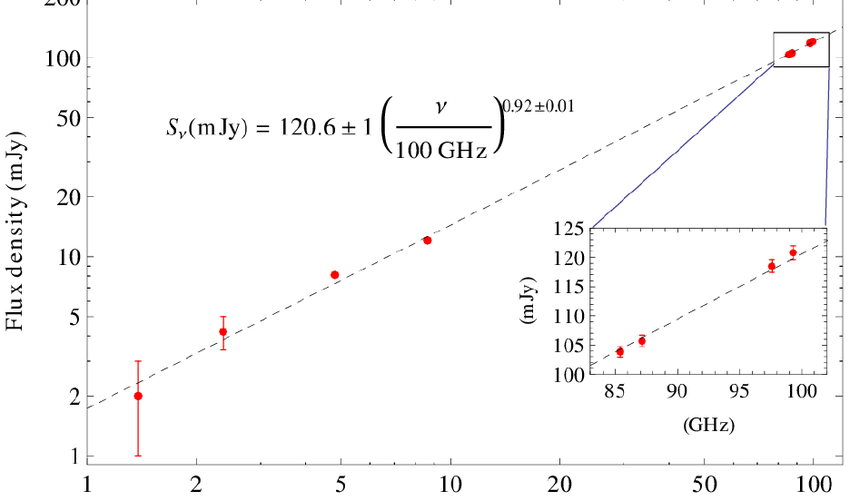TCARES is a 501(C)(3) non-profit.# How do we use the decibel in the design of our stations?

## Decibels: Pesky Little Critters

In our telecommunication careers, we may have all used dBs in our work. Here is a little background and how we use them in amateur radio. Decibels are a form of mathematical shorthand using logarithms. Logarithms probably originated with the ancient Greeks. Logarithms have the property expressing large values or ratios in more easily understood human terms.

Decibels originated when the engineering of analog telephone circuit became more complicated. The Bell System of fond memory in the 1920’s needed a way to design telephone circuits taking into account cable and component losses plus the gain of amplifiers. One of the first attempts was to use a standard mile of cable as a reference. They might have measured the loss at 1,000 cycles on a cable one mile long. So they could say a telephone circuit was short or long depending on the circuit parameters and how it measured.

Maybe not coincidentally, the loss of the standard cable mile was about what we would call now one decibel. To honor Alexander G. Bell they named the unit after him or one “Bel”. The Bel is actually a quite large ratio, so to make it easier to understand, they divided the Bel into ten parts or a decibel (dB). You could have had a centi-bel or a hundred parts of a Bel but the decibel or a Bell divided by ten was a much more convenient unit. It matched the physical word more closely.

## The decibel or dB is a reference ratio.

It has no meaning other than as a mathematical ratio. It becomes meaningful when referenced to a standard value such as a milli-watt or dBm. It can either have a negative or a plus value. A standard reference in telephone work is zero dBm or one milli-watt. So 10 dBm is equivalent to 10 milli-watts, -10dBm is equivalent to a tenth of a  milli-watt, 30 dBm is equivalent to one watt and so on.. Many other references are possible such as dBW where zero dBW is equivalent to one watt.

As an example the 200 watt power output in your transceiver could be rated at 53 dBm or 23 dBW. The receive signal power at your antenna could be -73 dBm for an S-9 signal on the meter. That is a power difference of 120 dB or a trillion times. Sounds like part of the national debt.

## How do we use the decibel in the design of our stations?

One example is the loss of coaxial cable. It usually expressed a loss of so many dB at 100 feet for a given frequency. The manufacturers provide lookup tables for their various types of cables. Antenna gain is given in dB. Using a reference to a dipole, the engineers would say the gain of a Yagi antenna as 6 dBd. The marketing department might show the antenna as 8.16 dBi. There the reference would be to an isotropic radiator. (does not exist in the real world).

Let us suppose our intrepid amateur radio operator wanted to seriously up grade his or her station on 20 meters. The current station is running 75 watts to a dipole up at about 30 feet. What would be the possible gain in dB using the original station as a reference by going to the legal limit of 1,500 watts and putting up a Yagi with 9 dBd gain (a very big antenna) at 90 feet! There are stations like that. Let’s add up the changes, so going from 75 to 1,500 watts is an increase of 13 dB, the Yagi with 9 dBd gain and the increase in height will give an additional 6dB (guess depends on terrain). So we have 13 plus 9 plus 6 equals 28 dB. The new station will be nearly 5 s-units (6 dB per s-unit) louder than our reference station.

The formulas for converting power ratios to dB and vice versa are given in the handbooks and online.

They look like this:

Convert power ratio to decibels: 10 * log(p1/p2) = decibels. Where p1 and p2 are the different power levels.

Convert decibels to power ratio:   10 raised to dB/10 = power ratio.  or 10^(dB/10)

Add up the decibels in a circuit taking into account whether the components are plus or minus. Amplifier and possible antenna gains are a plus and all the other stuff is minus.

Dig out that old calculator or spreadsheet with mathematical functions and work out a few examples, going from power ratios to dBs and back again.

So keep an eye out for the Pesky Little Critters called dBs, running around the shack. They do make sense and add up.